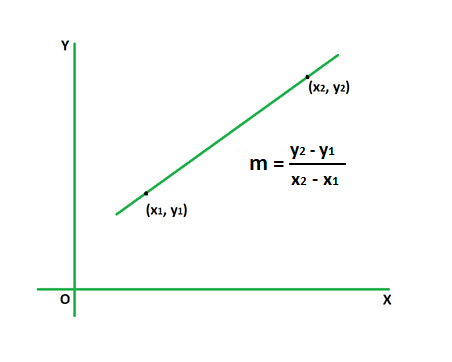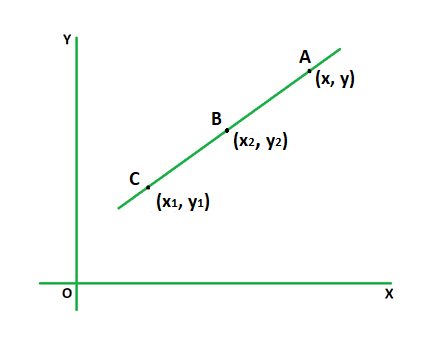GeeksforGeeks App
Open AppBrowser
Continue

# How to find the equation of a line from two points?

A line’s slope is a measure of its steepness and direction. It is defined as the change in y coordinate to the change in x coordinate of that line.  It is denoted by the symbol m. If two points (x1, y1) and (x2, y2) are connected by a straight line on a curve y = f(x), the slope is given by the ratio of the y-coordinate difference to x-coordinate difference.### How to find the equation of a line from two points?

Two-point form is used to find the equation of a line passing through two points. Its formula is given by,

y – y1 = m (x – x1)

orwhere,

m is the slope of line,

(x1, y1) and (x2, y2) are the two points through which line passes,

(x, y) is an arbitrary point on the line.

DerivationConsider a line with two fixed points B (x1, y1) and C (x2, y2). Another point A (x, y) is an arbitrary point on the line.

As the points A, B and C are concurrent the slope of AC must be equal to BC.

Using the formula for slope we get,

(y – y1) / (x – x1) = (y2 – y1) / (x2 – x1)

Multiplying both sides by (x – x1) we get,This derives the formula for two point form of a line.

### Sample Problems

Problem 1. Find the equation of a line passing through the points (2, 4) and (-1, 2).

Solution:

We have,

(x1, y1) = (2, 4)

(x2, y2) = (-1, 2)

Find the slope of the line.

m = (2 – 4)/(-1 – 2)

= -2/-3

= 2/3

Using the two point form we get,

y – y1 = m (x – x1)

y – 4 = 2/3 (x – 2)

3y – 12 = 2 (x – 2)

3y – 12 = 2x – 4

2x – 3y + 8 = 0

Problem 2. Find the equation of a line passing through the points (4, 5) and (3, 1).

Solution:

We have,

(x1, y1) = (4, 5)

(x2, y2) = (3, 1)

Find the slope of the line.

m = (1 – 5)/(3 – 4)

= -4/-1

= 4

Using the two point form we get,

y – y1 = m (x – x1)

y – 5 = 4 (x – 4)

y – 5 = 4x – 16

4x – y – 11 = 0

Problem 3. Find the equation of a line passing through the points (2, 1) and (4, 0).

Solution:

We have,

(x1, y1) = (2, 1)

(x2, y2) = (4, 0)

Find the slope of the line.

m = (0 – 1)/(4 – 2)

= -1/2

Using the two point form we get,

y – y1 = m (x – x1)

y – 1 = (-1/2) (x – 2)

2y – 2 = 2 – x

x + 2y – 4 = 0

Problem 4. Find the y-intercept of the equation of a line passing through the points (3, 5) and (8, 7).

Solution:

We have,

(x1, y1) = (3, 5)

(x2, y2) = (8, 7)

Find the slope of the line.

m = (7 – 5)/(8 – 3)

= 2/5

Using the two point form we get,

y – y1 = m (x – x1)

y – 5 = (2/5) (x – 3)

5y – 25 = 2x – 6

2x – 5y + 19 = 0

Put x = 0 to get the y-intercept.

=> 2 (0) – 5y + 19 = 0

=> 5y = 19

=> y = 19/5

Problem 5. Find the x-intercept of the equation of a line passing through the points (4, 8) and (1, 3).

Solution:

We have,

(x1, y1) = (4, 8)

(x2, y2) = (1, 3)

Find the slope of the line.

m = (3 – 8)/(1 – 4)

= -5/-3

= 5/3

Using the two point form we get,

y – y1 = m (x – x1)

y – 8 = (5/3) (x – 4)

3y – 24 = 5x – 20

5x – 3y + 4 = 0

Put y = 0 to get the x-intercept.

=> 5x – 3 (0) + 4 = 0

=> 5x + 4 = 0

=> x = -4/5

Problem 6. Find the slope of a line passing through the points (2, 7) and (-4, 5).

Solution:

We have,

(x, y) = (2, 7)

(x1, y1) = (-4, 5)

Using the formula we get,

y – y1 = m (x – x1)

=> 7 – 5 = m (2 – (-4))

=> 2 = m (2 + 4)

=> 6m = 2

=> m = 1/3

Problem 7. Find the slope of a line passing through the points (4, -5) and (6, 7).

Solution:

We have,

(x, y) = (4, -5)

(x1, y1) = (6, 7)

Using the formula we get,

y – y1 = m (x – x1)

=> -5 – 7 = m (4 – 6)

=> -12 = m (-2)

=> -2m = -12

=> m = 6

My Personal Notes arrow_drop_up
Related Tutorials